# How to Calculate and Solve for Standing Gas Solubility of a Fluid | The Calculator Encyclopedia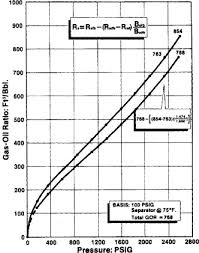The image above represents standing gas solubility.

To compute for the standing gas solubility, three essential parameters are needed and these are Solution Gas Specific Density (γg), System Pressure (P) and Standing Gas Solubility Parameter (x).

The formula for calculating the standing gas solubility:

Standing Rs = γg[(P / 18.2 + 1.4) 10x ]1.2048

Where:

Standing Rs = Standing Gas Solubility
γg = Solution Gas Specific Density
P = System Pressure
x = Standing Gas Solubility Parameter

Let’s solve an example;
Find the standing gas solubility when the solution gas specific density is 12, the system pressure is 40 and the standing gas solubility parameter is 20.

This implies that;

γg = Solution Gas Specific Density = 12
P = System Pressure = 40
x = Standing Gas Solubility Parameter = 20

Standing Rs = γg[(P / 18.2 + 1.4) 10x ]1.2048
Standing Rs = 12[(40 / 18.2 + 1.4) 1020 ]1.2048
Standing Rs = 12[(3.597802197802198) 1020]1.2048
Standing Rs = 12[(3.597802197802198) (100000000000000000000)]1.2048
Standing Rs = 121.2048
Standing Rs = 12[5.833292021408776e+24]
Standing Rs = 6.999950425690531e+25

Therefore, the standing gas solubility is 6.999950425690531e+25.

Nickzom Calculator – The Calculator Encyclopedia is capable of calculating the standing gas solubility.

To get the answer and workings of the standing gas solubility using the Nickzom Calculator – The Calculator Encyclopedia. First, you need to obtain the app.

You can get this app via any of these means:

To get access to the professional version via web, you need to register and subscribe for NGN 1,500 per annum to have utter access to all functionalities.
You can also try the demo version via https://www.nickzom.org/calculator

Android (Paid) – https://play.google.com/store/apps/details?id=org.nickzom.nickzomcalculator
Android (Free) – https://play.google.com/store/apps/details?id=com.nickzom.nickzomcalculator
Apple (Paid) – https://itunes.apple.com/us/app/nickzom-calculator/id1331162702?mt=8
Once, you have obtained the calculator encyclopedia app, proceed to the Calculator Map, then click on Petroleum under EngineeringNow, Click on Fluid Properties under Petroleum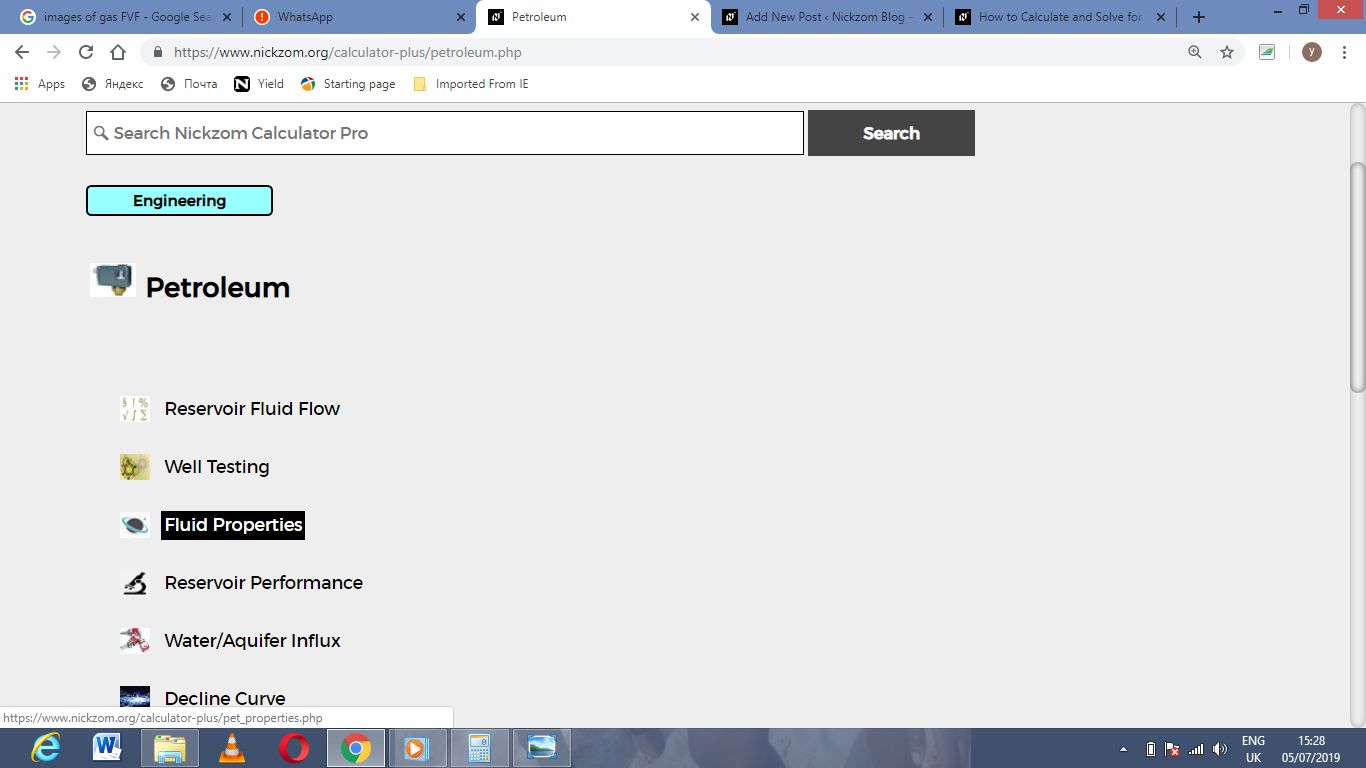Now, Click on Standing Gas Solubility under Fluid Properties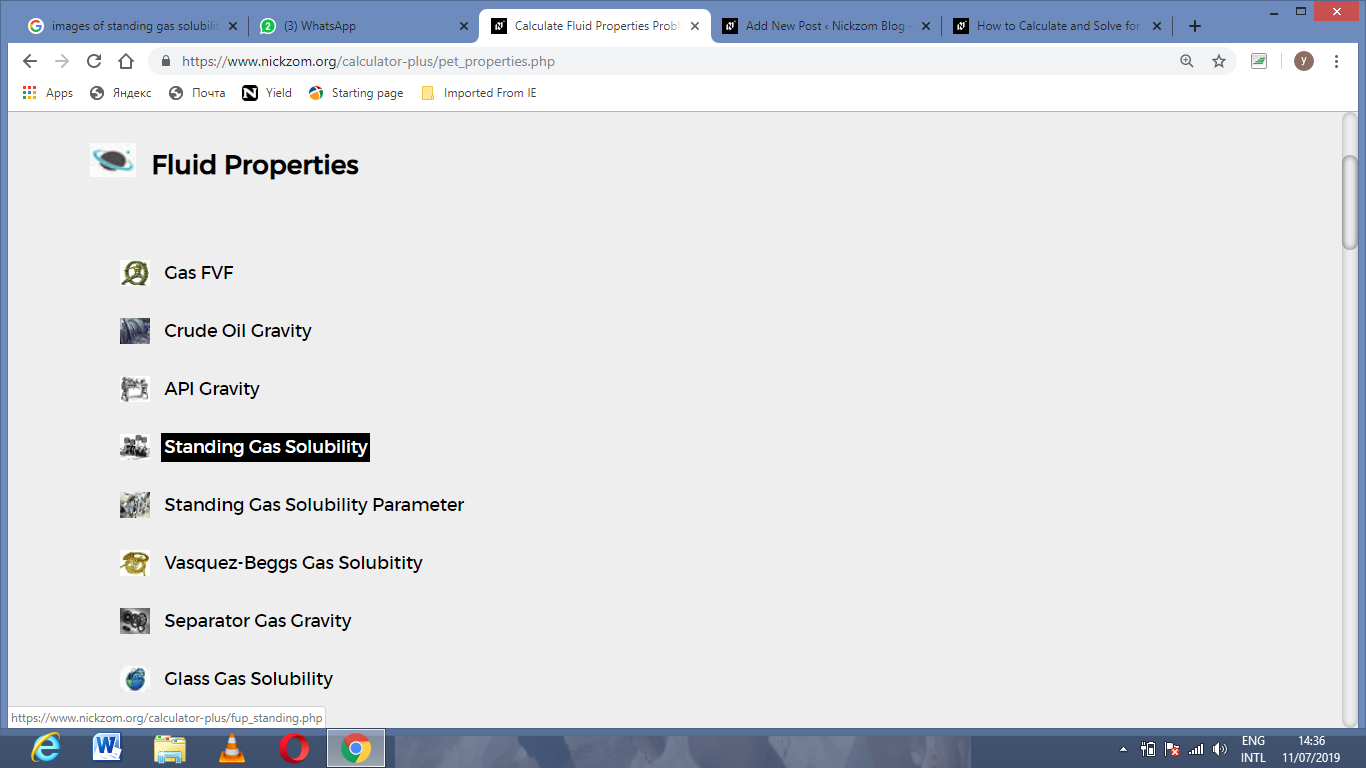The screenshot below displays the page or activity to enter your values, to get the answer for the standing gas solubility according to the respective parameters which are the Solution Gas Specific Density (γg), System Pressure (P) and Standing Gas Solubility Parameter (x).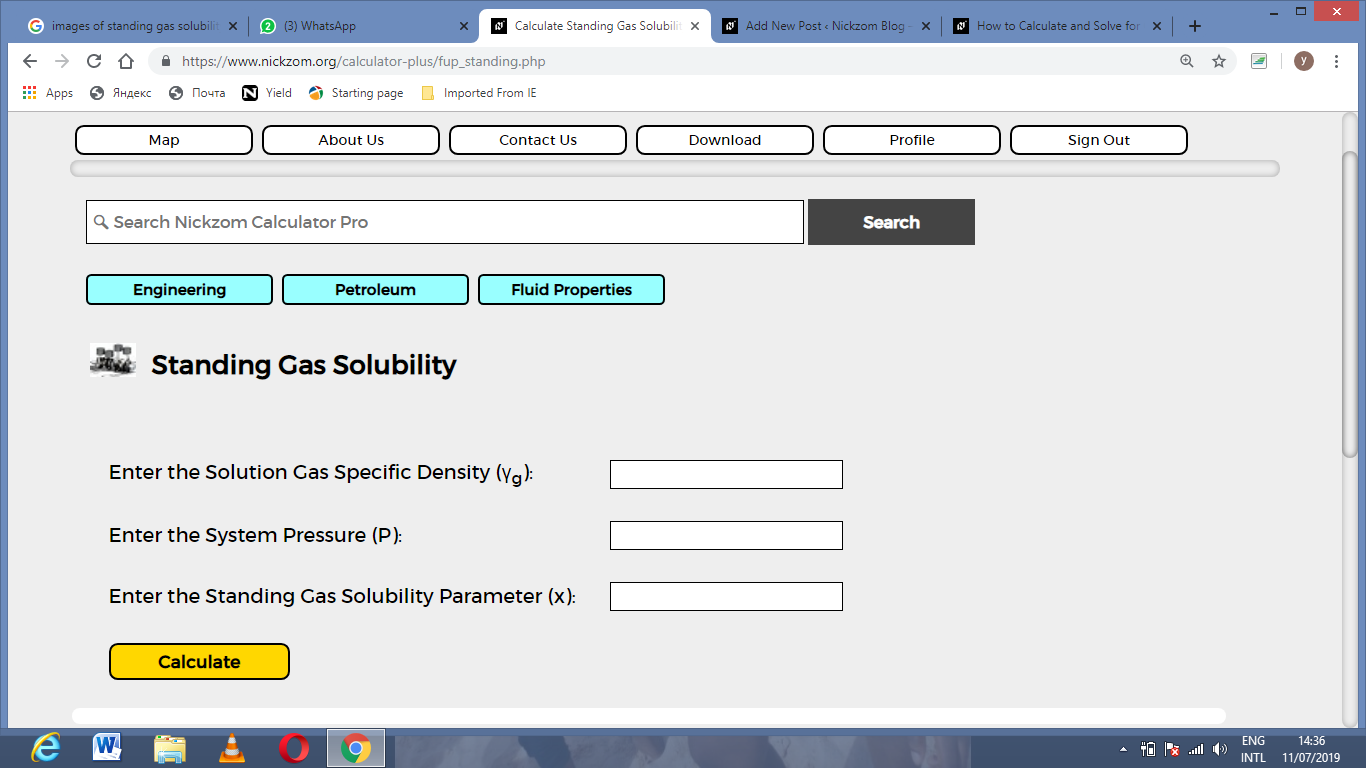Now, enter the values appropriately and accordingly for the parameters as required by the Solution Gas Specific Density (γg) is 12, System Pressure (P) is 40 and Standing Gas Solubility Parameter (x) is 20.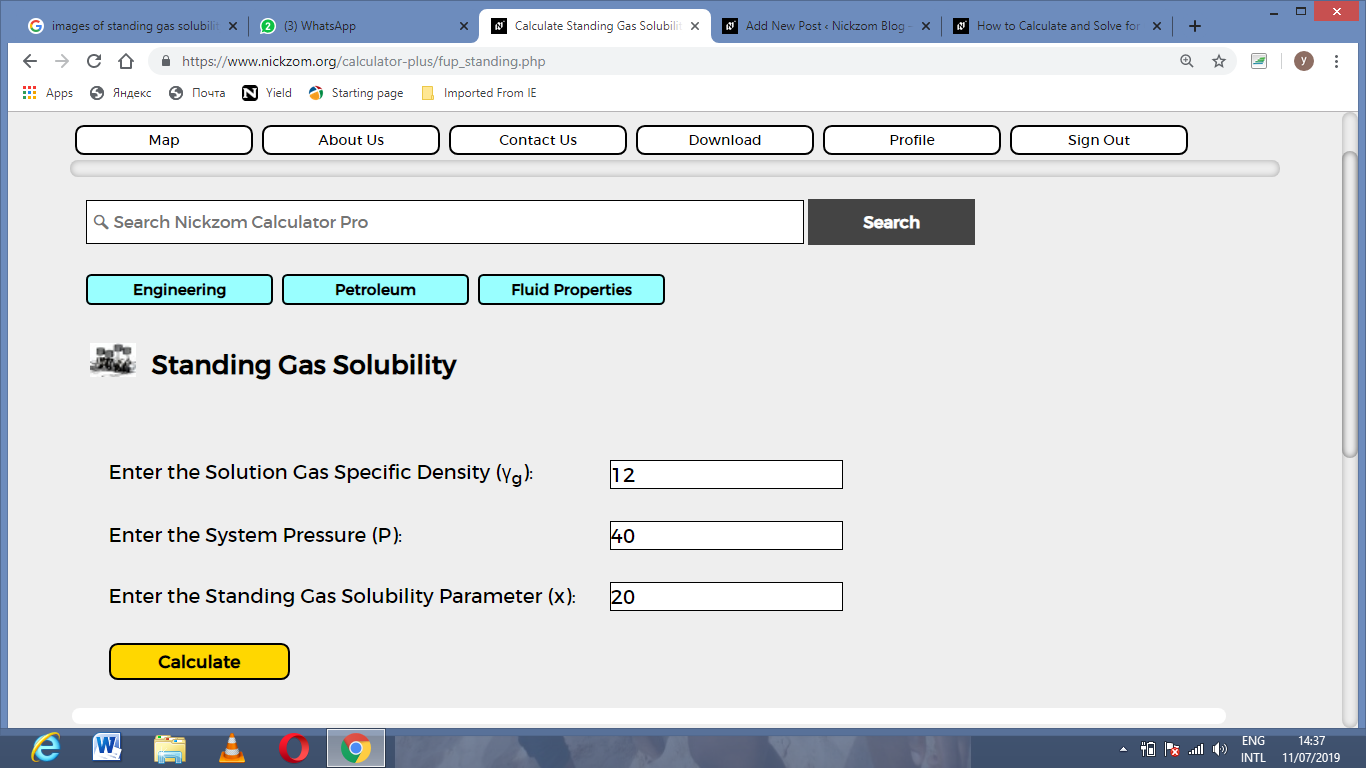Finally, Click on Calculate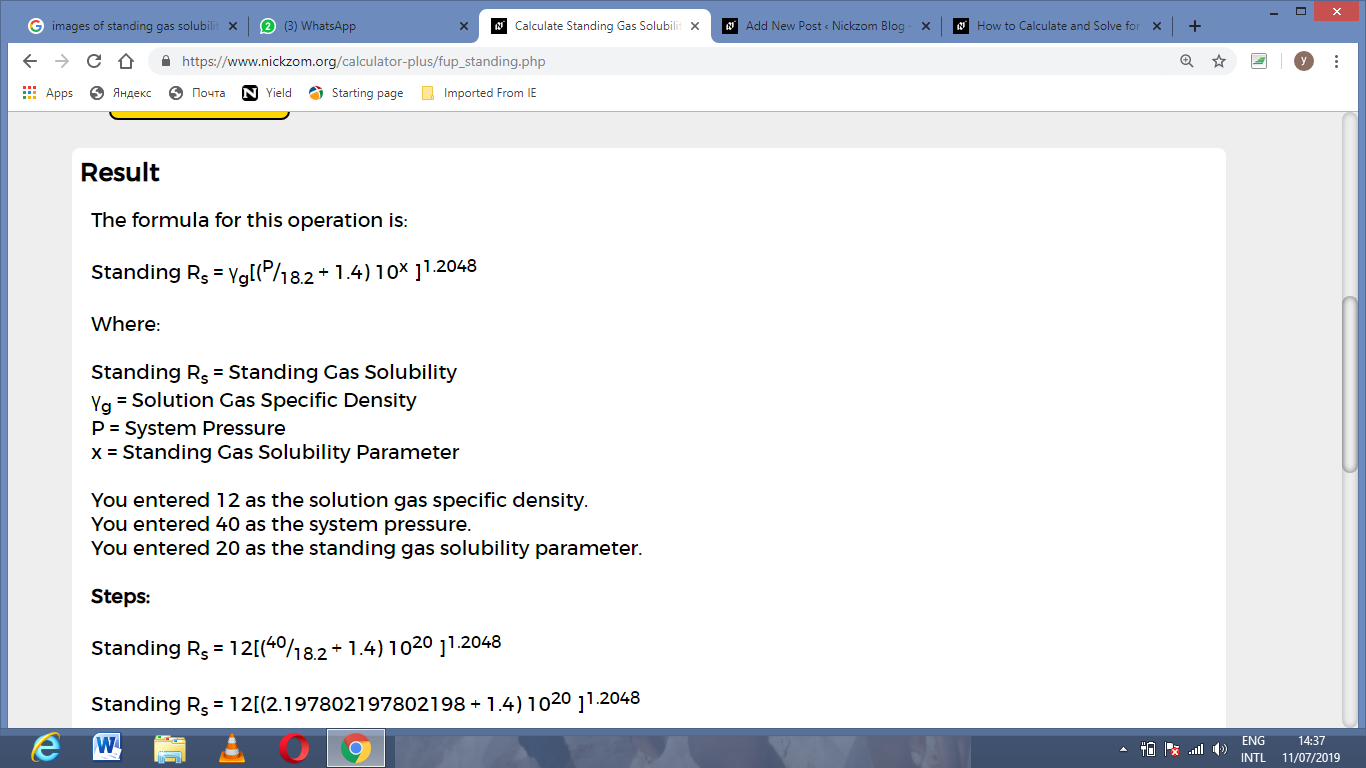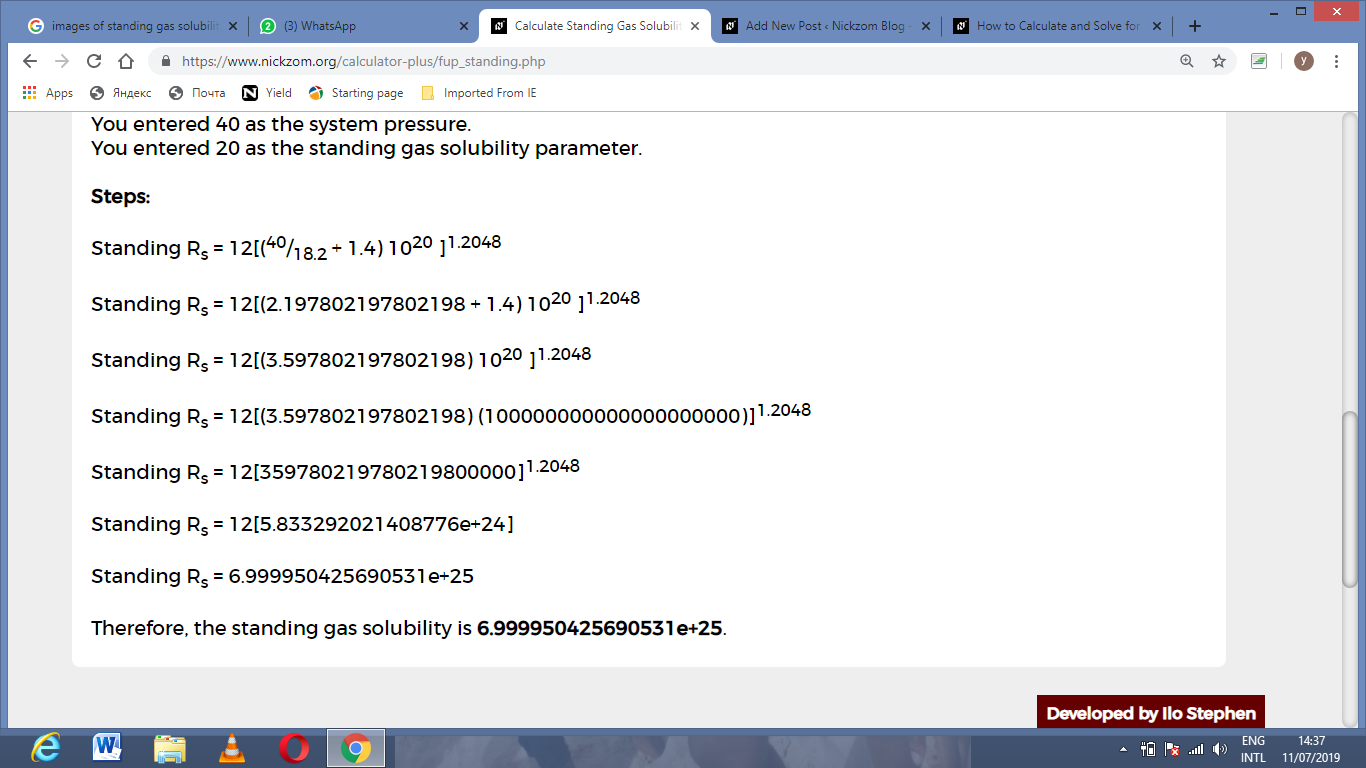As you can see from the screenshot above, Nickzom Calculator– The Calculator Encyclopedia solves for the standing gas solubility and presents the formula, workings and steps too.